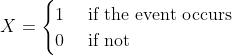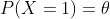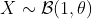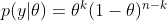# Bayesian estimator of the Bernoulli parameter

In this post, I will explain how to calculate a Bayesian estimator. The taken example is very simple: estimate the parameter θ of a Bernoulli distribution.

A random variable X which has the Bernoulli distribution is defined aswithIn this case, we can write.

In reality, the simplest way to eatimate θ is to sample X, count how many time the event occurs, then estimate the probability of occuring of event. This is exactly what the frequestists do.

In this post, I will show how do the Bayesian statisticians estimate θ. Although this doesn’t have a meaningful application, but it helps to understand how do the Bayesian statistics work. Let’s start.

## The posterior distribution of θ

Denote Y as the observation of the event. Given the parameter θ, if we sample the event n time, then the probability that the event occurs k time is (this is called the probability density function of Bernoulli )In Bayesian statistics, we would like to calculate

By using the Bayesian formula, we haveWith the prior distribution of theta as an Uniform distribution, p(θ) = 1, and it is easy to prove thatwhere Γ is the Gamma distribution. Hence, the posterior distribution isFortunately, this is the density function of the Beta distribution:We use the following properties for evaluating the posterior mean and variance of theta.

If, then## Simulation

In summary, the Bayesian estimator of theta is the Beta distribution with the  mean and variance as above. Here is the Python codes for simulating data and estimating theta

def bayes_estimator_bernoulli(data, a_prior=1, b_prior=1, alpha=0.05):
'''Input:
data is a numpy array with binary value, which has the distribution B(1,theta)    a_prior, b_prior: parameters of prior distribution Beta(a_prior, b_prior)    alpha: significant level of the posterior confidence interval for parameter    Model:
for estimating the parameter theta of a Bernoulli distribution    the prior distribution for theta is Beta(1,1)=Uniform[0,1]    Output:
a,b: two parameters of the posterior distribution Beta(a,b)
pos_mean: posterior estimation for the mean of theta
pos_var: posterior estimation for the var of theta'''
n = len(data)
k = sum(data)
a = k+1
b = n-k+1
pos_mean = 1.*a/(a+b)
pos_var = 1.*(a*b)/((a+b+1)*(a+b)**2)
## Posterior Confidence Interval
theta_inf, theta_sup = beta.interval(1-alpha,a,b)
print('Prior distribution: Beta(%3d, %3d)' %(a_prior,b_prior))
print('Number of trials: %d, number of successes: %d' %(n,k))
print('Posterior distribution: Beta(%3d,%3d)' %(a,b))
print('Posterior mean: %5.4f' %pos_mean)
print('Posterior variance: %5.8f' %pos_var)
print('Posterior std: %5.8f' %(np.sqrt(pos_var)))
print('Posterior Confidence Interval (%2.2f): [%5.4f, %5.4f]' %(1-alpha, theta_inf, theta_sup))
return a, b, pos_mean, pos_var

# Example
n = 129 # sample size
data = np.random.binomial(size=n, n=1, p=0.6)
a, b, pos_mean, pos_var = bayes_estimator_bernoulli(data)

And the result is

Prior distribution: Beta(  1,   1)
Number of trials: 129, number of successes: 76
Posterior distribution: Beta( 77, 54)
Posterior mean: 0.5878
Posterior variance: 0.00183556
Posterior std: 0.04284341
Posterior Confidence Interval (0.95): [0.5027, 0.6703]

In the simulation, we simulated 129 data from the Bernoulli distribution with θ=0.6. And the Bayesian estimation of θ is the posterior mean which is 0.5878.
This is a very simple example of Bayesian estimation. In reality, it is usually tricky to determinate a closed form solution of the posterior distribution from the given prior distribution. In that case, Monte Carlo technique is one of solutions to approximate the posterior distribution.
Please also check Gaussian Samples and N-gram language models for statistics knowledges.